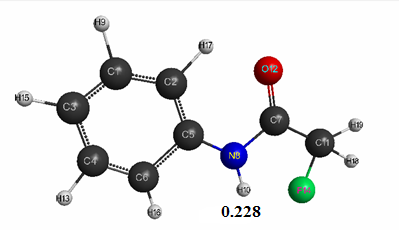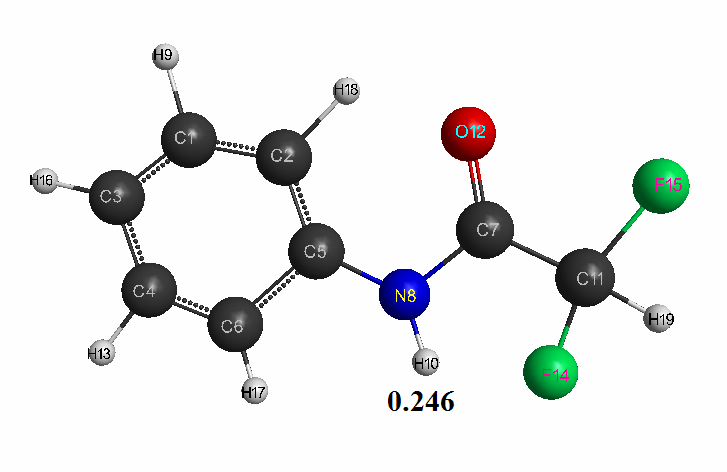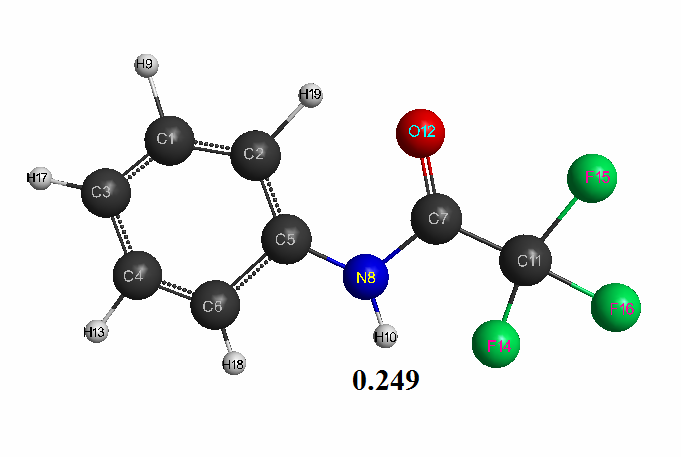The publication of the journal is supported by
SIA "P&M-Invest" Ltd and INEOS RAS
Volume # 5(126), September - October 2019 — "Quantum chemical calculation of some acetanilide molecules by the AM1 method"

DOI 10.17677/fn20714807.2019.05.01

Fluorine Notes, 2019, 126, 1-2

# QUANTUM CHEMICAL CALCULATION OF SOME ACETANILIDE MOLECULES BY THE AM1 METHOD

#### 1 Babkin V.A., 1 Vashuta Yu.A., 1 Andreev D.S., 1 Ignatov A.V., 1Bakholdin I.I. 2,3 Titova E.S., 2 Rakhimov A.I., 2 Rakhimova N.A., 2 Vo T.L.K., 2 Shishkin E.V., 4 Belousova V.S., 5 Denisyuk A.R., 6 Fomichev V.T., 7 K.Yu. Prochukhan

##### 7Bashkir State University, 450076, Republic of Bashkortostan, Ufa, Zaki Validi Street, 32

Abstract: Quantum chemical calculation of some acetanilide molecules: fluoroacetanilide, difluoroacetanilide and trifluoroacetanilide was performed using the AM1 method with geometry optimization for all parameters by the standard gradient method. The optimized geometrical and electronic structure of these compounds was obtained. Their acid strength was theoretically estimated (pKa = 9–12). It was established that the molecules belong to the class of medium H-acids (pKa <14).

Keywords: quantum-chemical calculation, AM1 method, fluoroacetanilide, difluoroacetanilide, trifluoroacetanilide, acid strength.

### Introduction and Purpose of the Work

Acetanilide serves as the starting compound in the synthesis of other organic intermediates, in the manufacture of dyes and medicine (for example, sulfamide drugs). It is also used to stabilize peroxide hydrogen and as plasticizer of cellulose nitrates [1-2]. Despite the fact that these compounds were discovered in the XIX century, so far the quantum chemical calculation of these compounds has not been performed. In this connection, the purpose of this work is the quantum chemical calculation of some acetanilide molecules: fluoroacetanilide, difluoroacetanilide and trifluoroacetanilide by the AM1 method with geometry optimization in all parameters using standard gradient method, built in the Firefly program , which is partially based on the GAMESS (US) source code , in the approximation of an isolated molecule in the gas phase, and a theoretical estimate of their acid strength. For the visual presentation of the molecule model, the famous program MacMolPlt was used .

### Calculation Results

Optimized geometric and electronic structure, total energy and electron energy of molecules of fluoroacetanilide, difluoroacetanilide and trifluoroacetanilide were obtained by the AM1 method and are shown in Fig. 1-3 and in Table 1-3. Using the AM1 formula for the method — рКа = 47.74 - 154.949 qmaxH+ (see Table 1), where qmaxH+ is the hydrogen atom maximum charge, pKa is the universal indicator of acidity, R is the correlation coefficient (0.98) , we find the value of acid strength of the molecules:

• Fluoroacetanilide: qmaxH+= +0,228, see Table. 1. pKa = 12.4.
• Difluoroacetanilide: qmaxH+= +0,246, see Table 2. pKa = 9.6.
• Trifluoroacetanilide: qmaxH+= +0,249, see Table 3. pKa = 9.1.

Thus, we carried out the quantum-chemical calculation of some acetanilide molecules (fluoroacetanilide, difluoroacetanilide and trifluoroacetanilide) using the AM1 method with geometry optimization in all parameters by the standard gradient method. The optimized geometrical and electronic structure of these compounds was obtained. Their acid strength was theoretically estimated (pKa = 9–12). It was established that these molecules belong to the class of medium H-acids (pKa <14). It should also be noted, that the theoretically calculated acid strength value of trifluoroacetanilide (pKa = 9.1) is in good agreement with the known experimental value of this compound (pKa = 9.51) .Figure 1. The geometric and electronic structure of the fluoroacetanilide molecule (Е0= -207101 kJ/mol)

Table 1. The optimized bond lengths, valent angles and atom charges of the fluoroacetanilide molecule, obtained by the AM1 method.Figure 2. The geometric and electronic structure of the difluoroacetanilide molecule (Е0= -252595 kJ/mol).

Table 2. The optimized bond lengths, valent angles and atom charges of the difluoroacetanilide molecule, obtained by the AM1 method.Figure 3. The geometric and electronic structure of the trifluoroacetanilide molecule(Е0= -298087 kJ/mol).

Table 3. The optimized bond lengths, valent angles and atom charges of the trifluoroacetanilide molecule, obtained by the AM1 method.Table 4. The total energy (Е0), the hydrogen atom maximum charge (qmaxH+), acid strength (pKa).

 No. Molecule Е0, kJ/mol qmaxH+ pKa 1 Fluoroacetanilide -207101 0.228 12.4 2 Difluoroacetanilide -252595 0.246 9.6 3 Trifluoroacetanilide -298087 0.249 9.1

### Literature

1. Acetanilide [Electronic resource]: From Wikipedia, the free encyclopedia: Version 92273930, saved at 6:12 UTC on April 23, 2018 / Wikipedia Authors // Wikipedia, the free encyclopedia. - Electron. - San Francisco: Wikimedia Foundation, 2018. - Access Mode: https://ru.wikipedia.org/?oldid=92273930 .
2. Chemical Encyclopedia. In five volumes. / Ch. ed. I. L. Knunyants. - M.: Soviet Encyclopedia, 1988. – vol. 1. – p. 225.
3. Alex A. Granovsky, Firefly version 8, www.http://classic.chem.msu.su/gran/firefly/index.html
4. M.W. Schmidt, K.K. Baldridge, J.A. Boatz, S.T. Elbert, M.S. Gordon, J.H. Jensen, S. Koseki, N. Matsunaga, K.A. Nguyen, S.J. Su, T.L. Windus, M. Dupuis, J.A. Montgomery. "General Atomic and Molecular Electronic Structure System". Journal of Computational Chemistry, vol. 14, 1347-1363 (1993). doi:10.1002/jcc.540141112
5. B.M. Bode, M.S. Gordon. "MacMolPlt: A graphical user interface for GAMESS". Journal of Molecular Graphics and Modelling, vol. 16, No. 3, 1998, p. 133-138.
6. Babkin V.A., Andreev D.S., Fomichev V.T., Zaikov G.E., Mukhamedzyanova E.R. On the correlation dependence of the universal acidity index with the maximum charge on the hydrogen atom of H-acids. The AM1 method. "Bulletin" of Kazan Technological University. 2012, vol. 15. No 8, p. 103-114.
7. General Organic Chemistry. // General ed. D. Barton and W. D. Ollis, -M.: Chemistry, 1983. -vol. 4, p.441.

ARTICLE INFO